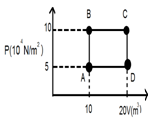# Thermodynamics - Free online Chemistry MCQ Test Series, Practice Questions with Solutions for JEE, NEET, Engineering and Medical Admission Tests

Chemistry Solved MCQ Test Series, Practice Questions
NEET, IIT JEE Main, AIIMS, NTSE

Welcome to your own site India Study Solution Below you will get carefully selected 10 MCQ practice questions in our each Test Series with hints & solutions (given at the end) from Chemistry Solutions chapter - Thermodynamics, Thermochemistry.
All these are typically important questions for NEET-UG, JEE Main & Advanced (IIT) AIIMS, JIPMER, COMEDK, MBBS Admission Tests, Engineering Entrance Exams, NEST and other such competitive exams.
Thermodynamics (Syllabus for NEET and JEE):
Fundamentals of thermodynamics: System and surroundings, extensive and intensive properties, state functions, types of processes. Thermal equilibrium, Zeroth law of thermodynamics, concept of temperature. First  law  of  thermodynamics: internal  energy  and  enthalpy,  heat  capacity  and  specific  heat, measurement of U and H, Hess’s law of constant heat summation. Enthalpies of bond dissociation, combustion, formation, atomization, sublimation, phase transition, ionization, solution and dilution. Introduction of entropy as state function. Second law of thermodynamics: Gibbs energy change for spontaneous and non-spontaneous processes, reversible and irreversible processes, criteria for equilibrium and spontaneity. Carnot engine and its efficiency. Third law of thermodynamics: Brief introduction.

### Chemistry Practice Questions: Thermodynamics (Thermochemistry)MCQ Test Series – Set 1 (Q. No. 1-10)

Question 1: Which of the following statements is correct?
a. Only internal energy is a state function but not work.
b. Only work is a state function but not internal energy.
c. Both internal energy and work are state functions.
d. Neither internal energy nor work is a state function.

Question 2: The amount of water that can be dissociated by the amount of heat liberated when 49 g H2SO4 and 20 g NaOH are mixed and reacted in aqueous solution is:
a. 1.803
b. 3.58
c. 0.895
d. 17.9Question 3: A sample of 2 kg of helium (assumed ideal) is taken through the process ABC and another sample of 2 kg of the same gas is taken through the process ADC. Then the temperature of the states A and B are (Given R = 8.3 joules/mol K):

a. TA = 120.5K, TB = 120.5K
b. TA = 241 K, TB = 241 K
c. TA = 120.5 K, TB = 241 K
d. TA = 241 K, TB = 482 K

Question 4: What is the work done against the atmosphere when 25 grams of water vaporises at 373 K against a constant external pressure of atm ? Assume that steam obeys perfect gas laws. Given that the molar enthalpy of vaporization is 9.72 kcal/mole, what is the change of internal energy in the above process?
a. 1294.0 cals, 11247 cals
b. 921.4 cals, 11074 cals
c. 1024.8 cals, 12470.6 cals
d. 1129.3 cals, 10207 cals

Question 5: Warming ammonium chloride with sodium hydroxide in a test tube is an example of:
a. Closed system
b. Isolated system
c. Open system
d. None of these

a. Q = 0
b. ∆E = 0
c. ∆W = 0
d. ∆V = 0

Question 7: A gas expands from 10 litres to 20 litres against a constant external pressure of 10 atm. The pressure-volume work done by the system is
a. 100 L atm
b. -100 L atm
c. 10 L atm
d. -10 L atm

Question 8: In an isothermal expansion of a gaseous sample the correct relation is (consider w (work) with sign according to new IUPAC convention)
[The reversible and irreversible processes are carried out between same initial and final states]
a. wrev  > wirrev
b. wirrev  > wrev
c. qrev< qirrev
d. cannot be predicted

Question 9: A tightly closed thermoflask contains some ice cubes. This constitutes
a. closed system
b. open system
c. isolated system
d. Non-thermodynamic system

Question 10: The process CO2 (g) + H2O → 2H+ (aq) + CO3-2 (aq),
∆H = +2000J taking place in a closed vessel is accompanied with [given S0 of CO2, H2O, H+ (aq) & CO3-2(aq) = 213, 70, 0 & 57J/Mol/K]
a. DSsys = -340 J/K
b. DSsur ≈ -7KJ/K
c. DStotal = -333 J/K
d. DSsys = -340 J/K

India Study Solution - Hints and Solutions Chemistry MCQ Test Series
Thermodynamics
Solutions of Chemistry Test Series (MCQ) – Set 1 (Q. No.1 –10)
T2 = 4T­­1
PA VA = nRTA
5 x 10 = [(2 x 1000) ÷ 2] x 0.082 TA
or, TA = 120.5,
at constant volume, (PA / TA) = (PB / TB)
TB = PB.TA / TA = 241 K.
Mole of H2O = 1.39
Pv = nRT
1 x v = 1.39 x 0.082 x 373
v = 42.8.
w = Pext. dv = 1 x [42.8] atm x lit
= (– 42.8 x 101.325) J = [–(42.8 x 101.325) ÷ 4.2] cal
= 1024.8 cal
H = E + (Pv) = 12470.6 cal/s.